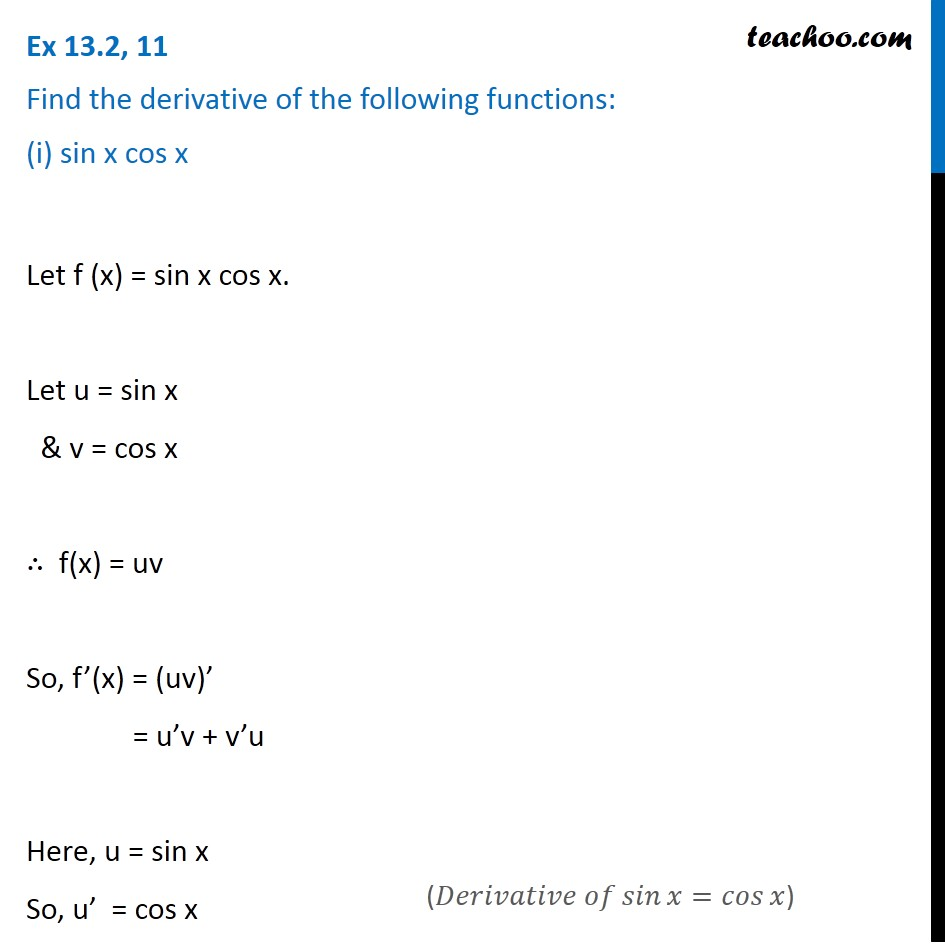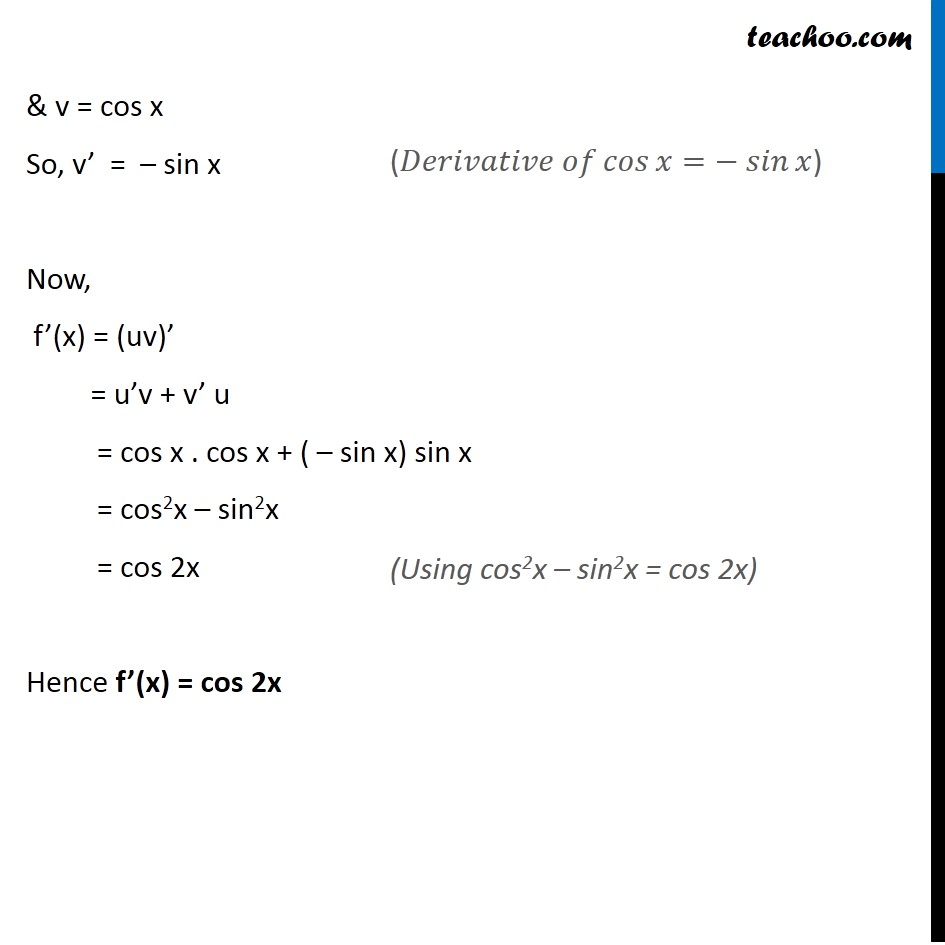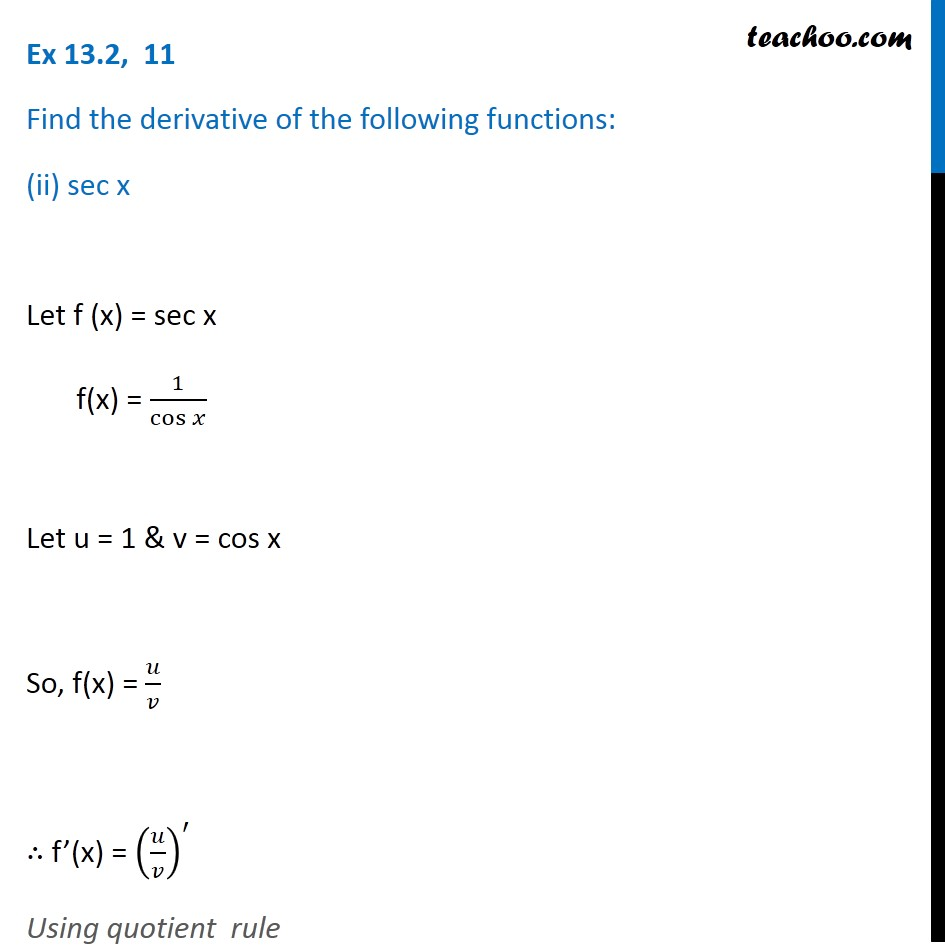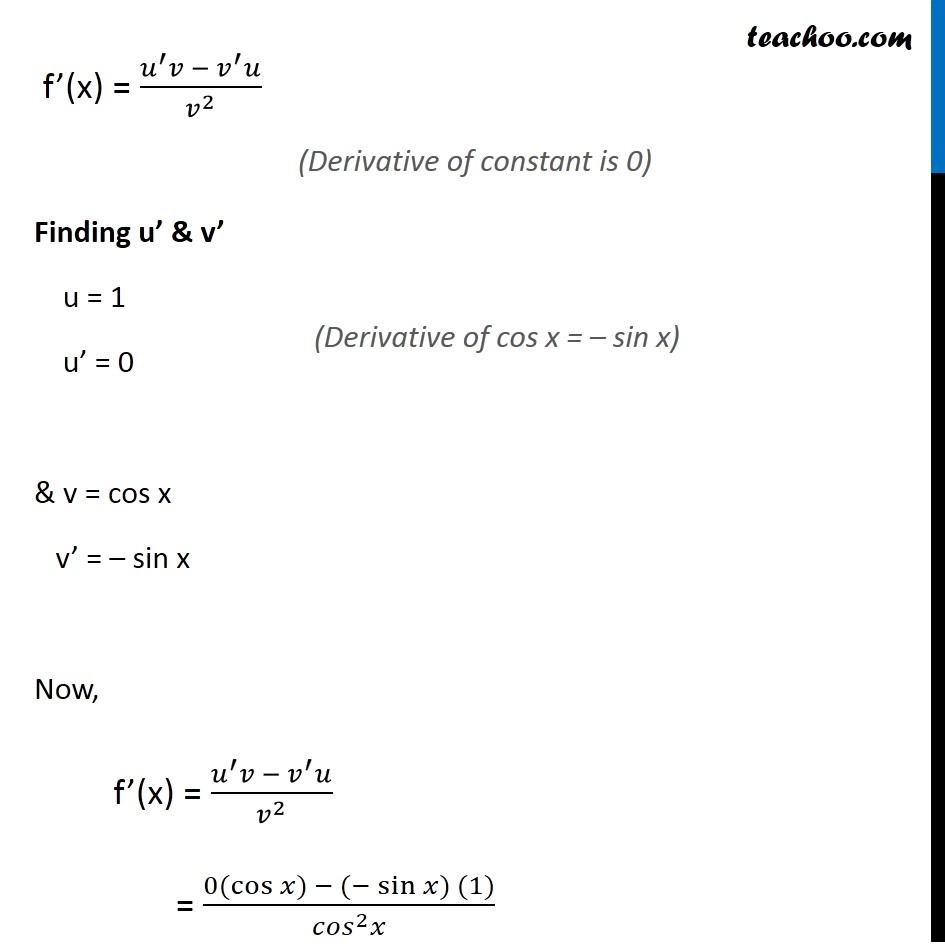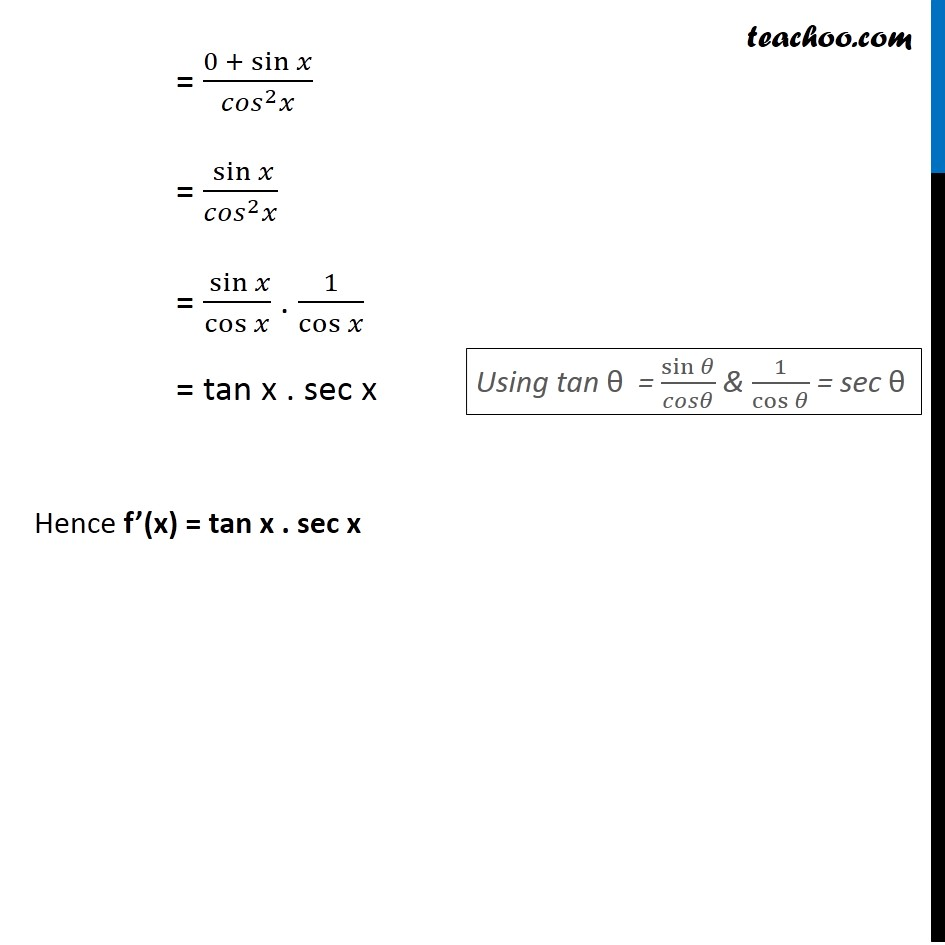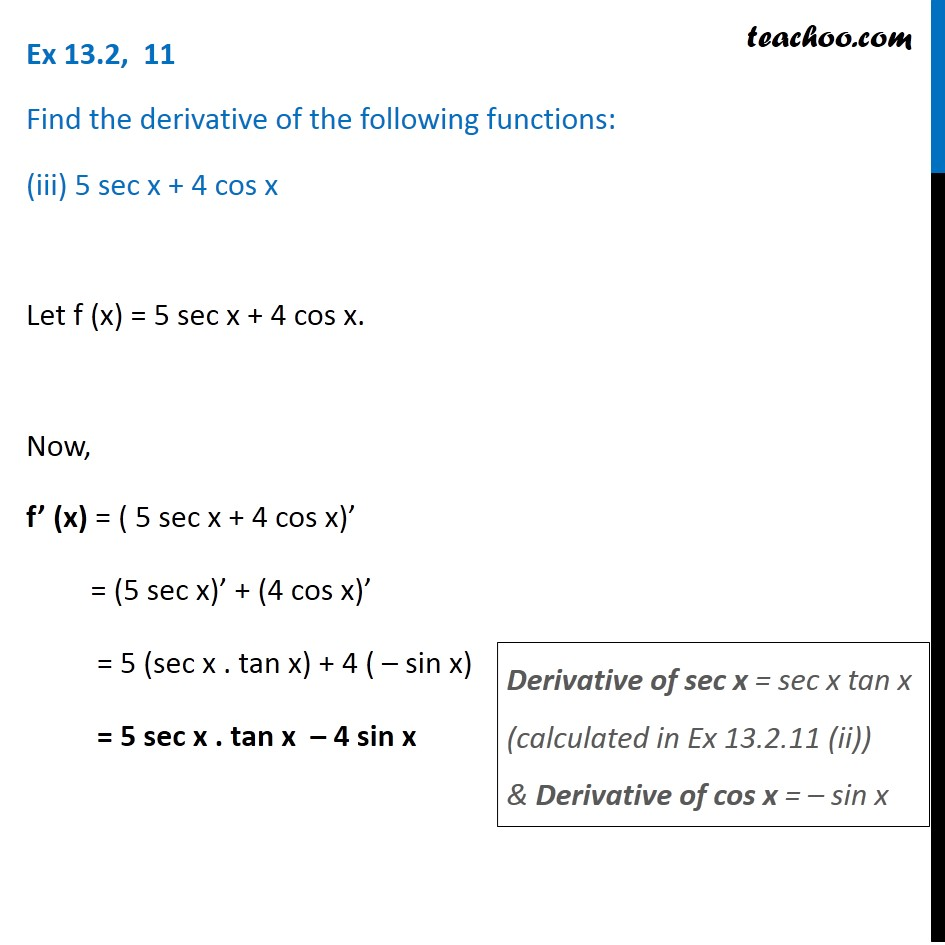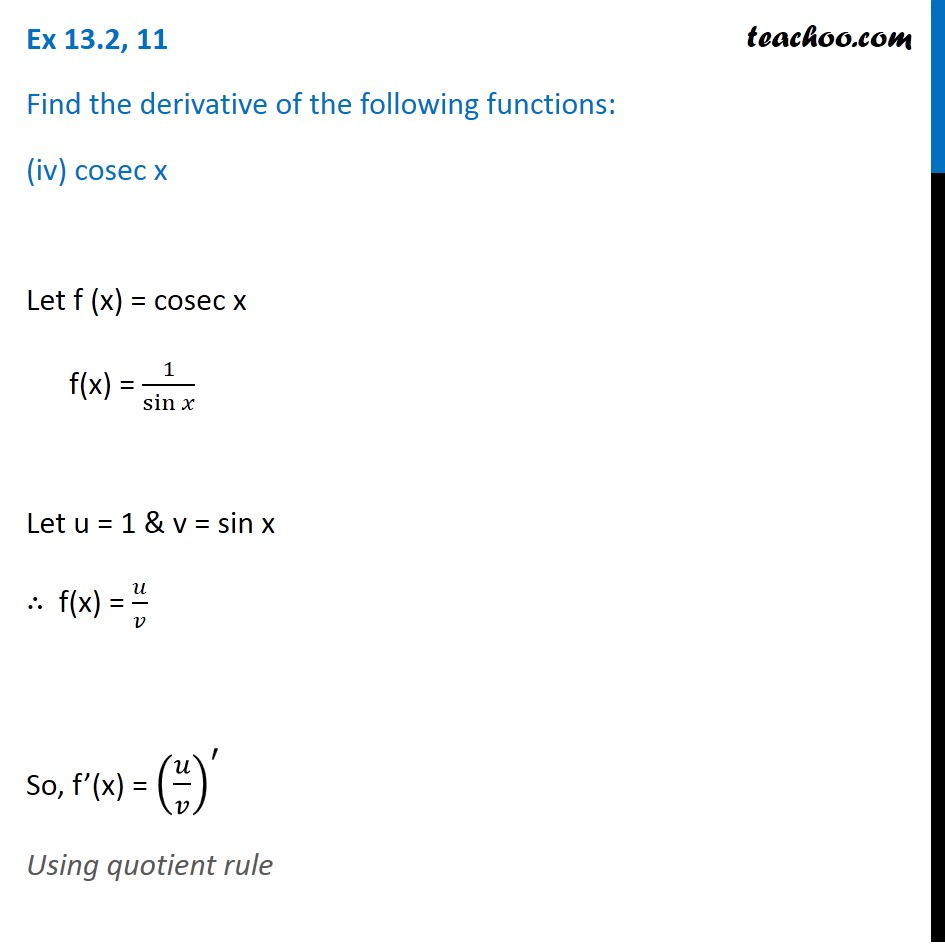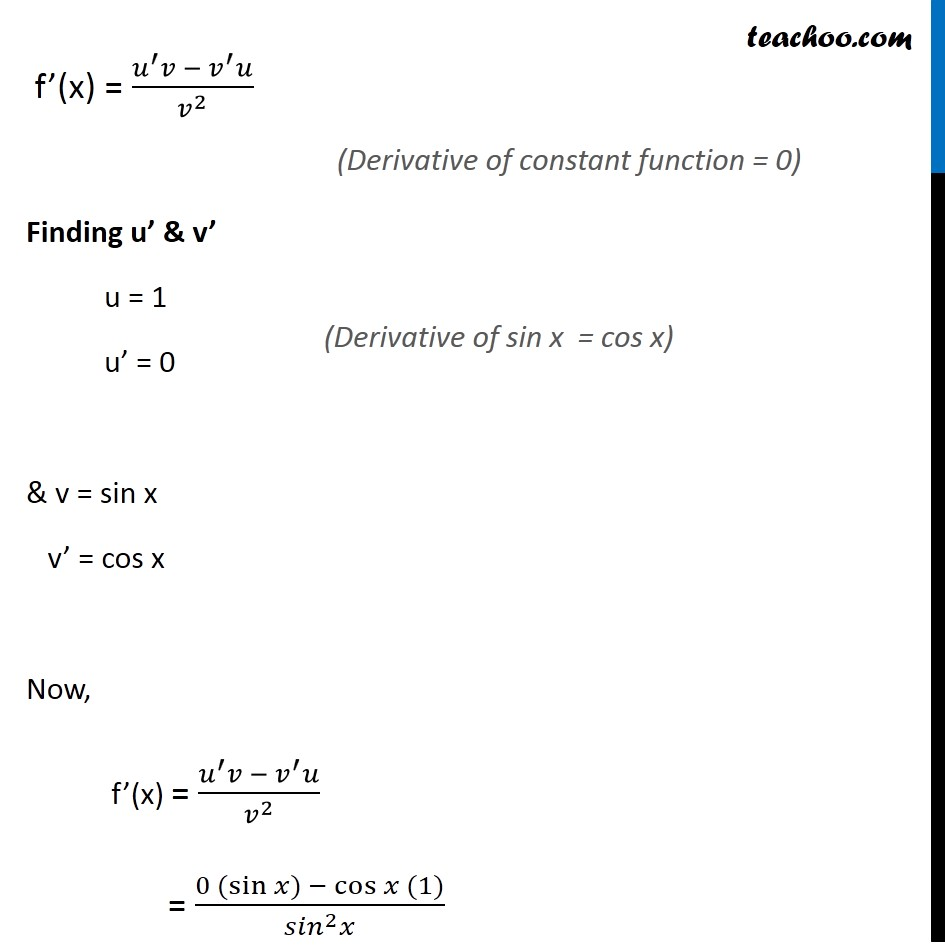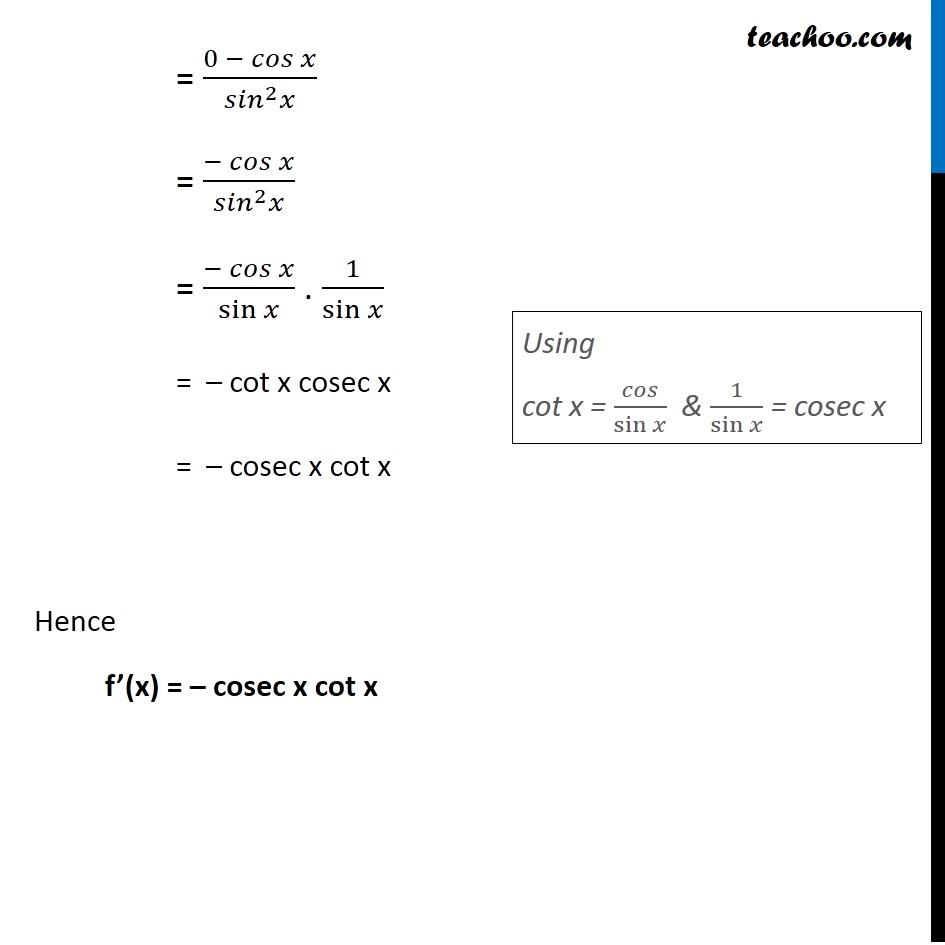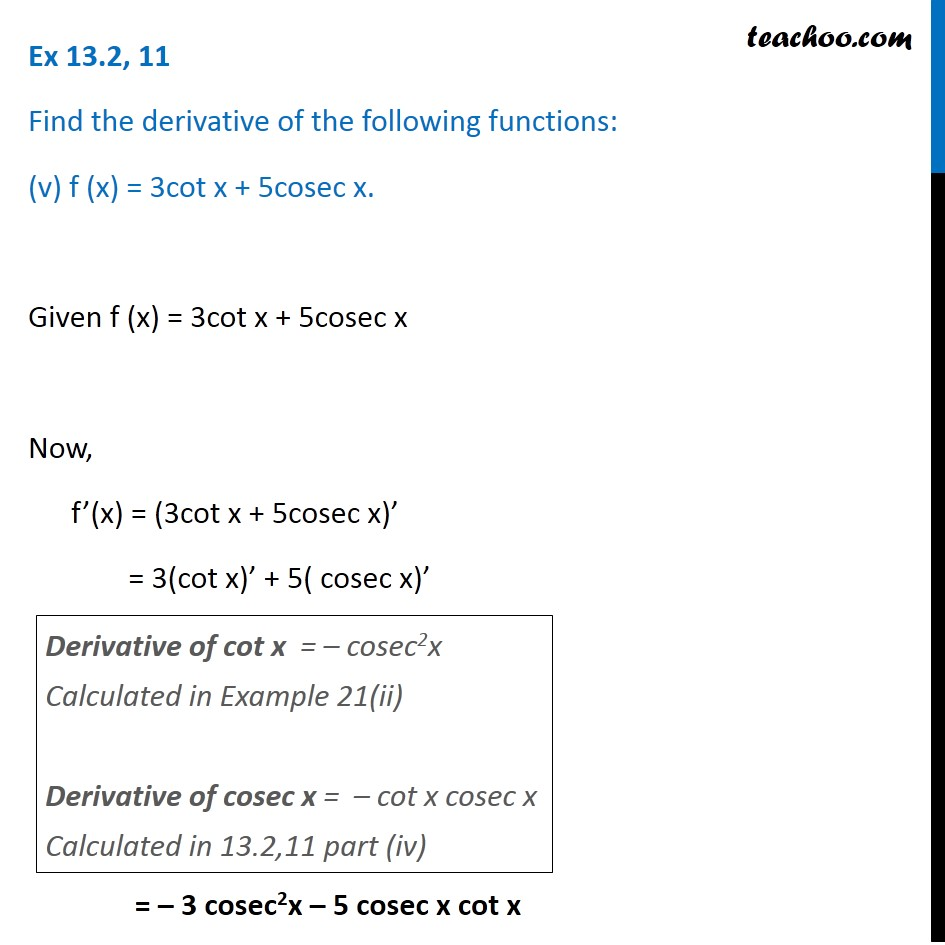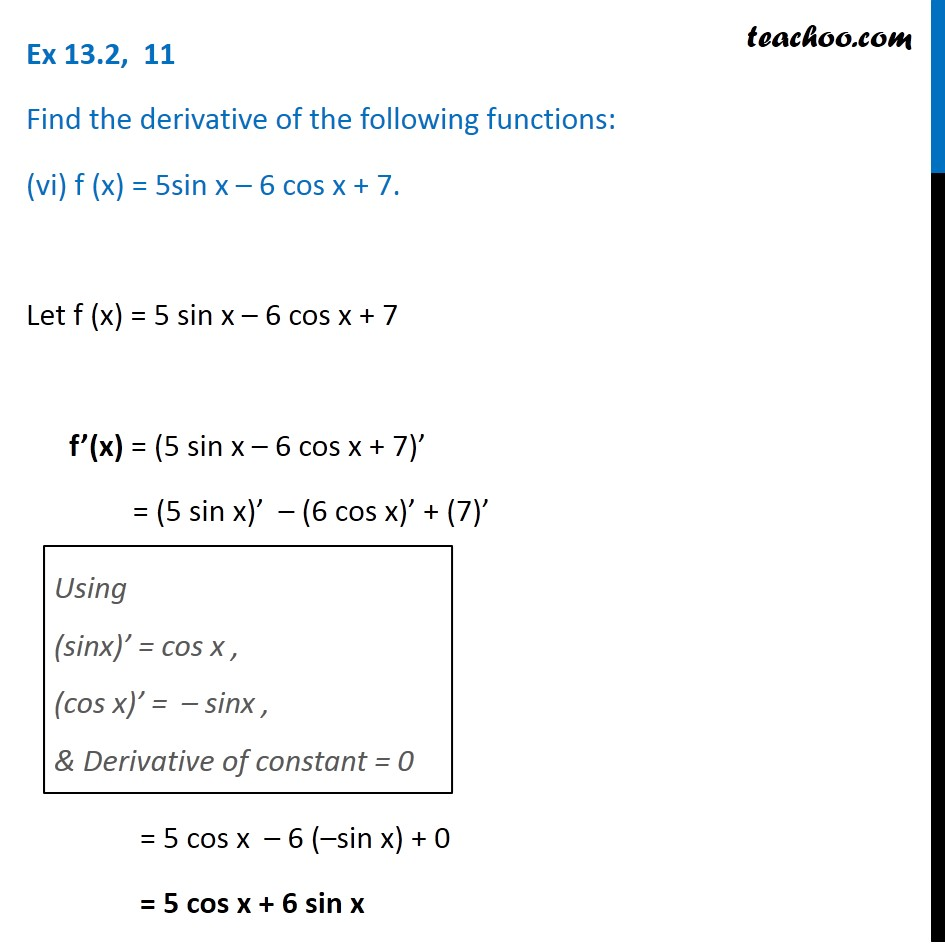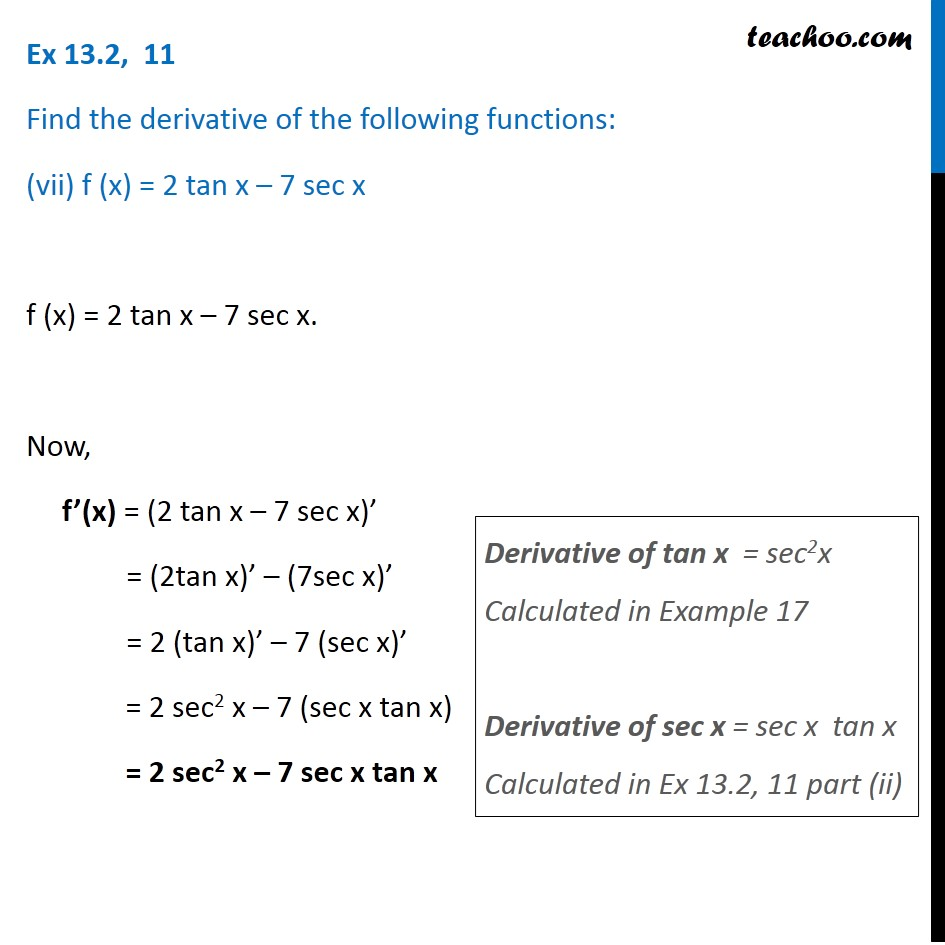1. Chapter 13 Class 11 Limits and Derivatives
2. Serial order wise
3. Ex 13.2

Transcript

Ex 13.2, 11 Find the derivative of the following functions: (i) sin x cos x Let f (x) = sin x cos x. Let u = sin x & v = cos x ∴ f(x) = uv So, f’(x) = (uv)’ = u’v + v’u Here, u = sin x So, u’ = cos x (𝐷𝑒𝑟𝑖𝑣𝑎𝑡𝑖𝑣𝑒 𝑜𝑓 𝑠𝑖𝑛⁡〖𝑥=𝑐𝑜𝑠⁡𝑥 〗) & v = cos x So, v’ = – sin x Now, f’(x) = (uv)’ = u’v + v’ u = cos x . cos x + ( – sin x) sin x = cos2x – sin2x = cos 2x Hence f’(x) = cos 2x (𝐷𝑒𝑟𝑖𝑣𝑎𝑡𝑖𝑣𝑒 𝑜𝑓 𝑐𝑜𝑠⁡〖𝑥=〖− 𝑠𝑖𝑛〗⁡𝑥 〗) (𝐷𝑒𝑟𝑖𝑣𝑎𝑡𝑖𝑣𝑒 𝑜𝑓 𝑐𝑜𝑠⁡〖𝑥=〖− 𝑠𝑖𝑛〗⁡𝑥 〗) Ex 13.2, 11 Find the derivative of the following functions: (ii) sec x Let f (x) = sec x f(x) = 1/cos⁡𝑥 Let u = 1 & v = cos x So, f(x) = 𝑢/𝑣 ∴ f’(x) = (𝑢/𝑣)^′ Using quotient rule f’(x) = (𝑢^′ 𝑣 − 𝑣^′ 𝑢)/𝑣^2 Finding u’ & v’ u = 1 u’ = 0 & v = cos x v’ = – sin x Now, f’(x) = (𝑢^′ 𝑣 − 𝑣^′ 𝑢)/𝑣^2 = (0(cos⁡〖𝑥) − (−sin⁡〖𝑥) (1)〗 〗)/(〖𝑐𝑜𝑠〗^2 𝑥) (Derivative of constant is 0) (Derivative of cos x = – sin x) = (0 +〖 sin〗⁡𝑥)/(〖𝑐𝑜𝑠〗^2 𝑥) = 〖 sin〗⁡𝑥/(〖𝑐𝑜𝑠〗^2 𝑥) = 〖 sin〗⁡𝑥/cos⁡𝑥 . 1/cos⁡𝑥 = tan x . sec x Hence f’(x) = tan x . sec x Using tan θ = sin⁡𝜃/𝑐𝑜𝑠𝜃 & 1/cos⁡𝜃 = sec θ Ex 13.2, 11 Find the derivative of the following functions: (iii) 5 sec x + 4 cos x Let f (x) = 5 sec x + 4 cos x. Now, f’ (x) = ( 5 sec x + 4 cos x)’ = (5 sec x)’ + (4 cos x)’ = 5 (sec x . tan x) + 4 ( – sin x) = 5 sec x . tan x – 4 sin x Derivative of sec x = sec x tan x (calculated in Ex 13.2.11 (ii)) & Derivative of cos x = – sin x Ex 13.2, 11 Find the derivative of the following functions: (iv) cosec x Let f (x) = cosec x f(x) = 1/sin⁡𝑥 Let u = 1 & v = sin x ∴ f(x) = 𝑢/𝑣 So, f’(x) = (𝑢/𝑣)^′ Using quotient rule f’(x) = (𝑢^′ 𝑣 −〖 𝑣〗^′ 𝑢)/𝑣^2 Finding u’ & v’ u = 1 u’ = 0 & v = sin x v’ = cos x Now, f’(x) = (𝑢^′ 𝑣 −〖 𝑣〗^′ 𝑢)/𝑣^2 = (0 (sin⁡〖𝑥) −〖 cos〗⁡〖𝑥 (1)〗 〗)/(〖𝑠𝑖𝑛〗^2 𝑥) (Derivative of constant function = 0) (Derivative of sin x = cos x) = (0 − 𝑐𝑜𝑠 𝑥)/(〖𝑠𝑖𝑛〗^2 𝑥) = (− 𝑐𝑜𝑠 𝑥)/(〖𝑠𝑖𝑛〗^2 𝑥) = (− 𝑐𝑜𝑠 𝑥)/sin⁡𝑥 . 1/sin⁡𝑥 = – cot x cosec x = – cosec x cot x Hence f’(x) = – cosec x cot x Using cot x = 𝑐𝑜𝑠/sin⁡𝑥 & 1/sin⁡𝑥 = cosec x Ex 13.2, 11 Find the derivative of the following functions: (v) f (x) = 3cot x + 5cosec x. Given f (x) = 3cot x + 5cosec x Now, f’(x) = (3cot x + 5cosec x)’ = 3(cot x)’ + 5( cosec x)’ = – 3 cosec2x – 5 cosec x cot x Derivative of cot x = – cosec2x Calculated in Example 21(ii) Derivative of cosec x = – cot x cosec x Calculated in 13.2,11 part (iv) Ex 13.2, 11 Find the derivative of the following functions: (vi) f (x) = 5sin x – 6 cos x + 7. Let f (x) = 5 sin x – 6 cos x + 7 f’(x) = (5 sin x – 6 cos x + 7)’ = (5 sin x)’ – (6 cos x)’ + (7)’ Using (sinx)’ = cos x , (cos x)’ = – sinx , & Derivative of constant = 0 = 5 cos x – 6 (–sin x) + 0 = 5 cos x + 6 sin x Ex 13.2, 11 Find the derivative of the following functions: (vii) f (x) = 2 tan x – 7 sec x f (x) = 2 tan x – 7 sec x. Now, f’(x) = (2 tan x – 7 sec x)’ = (2tan x)’ – (7sec x)’ = 2 (tan x)’ – 7 (sec x)’ = 2 sec2 x – 7 (sec x tan x) = 2 sec2 x – 7 sec x tan x Derivative of tan x = sec2x Calculated in Example 17 Derivative of sec x = sec x tan x Calculated in Ex 13.2, 11 part (ii)

Ex 13.2

About the AuthorDavneet Singh
Davneet Singh is a graduate from Indian Institute of Technology, Kanpur. He has been teaching from the past 10 years. He provides courses for Maths and Science at Teachoo.